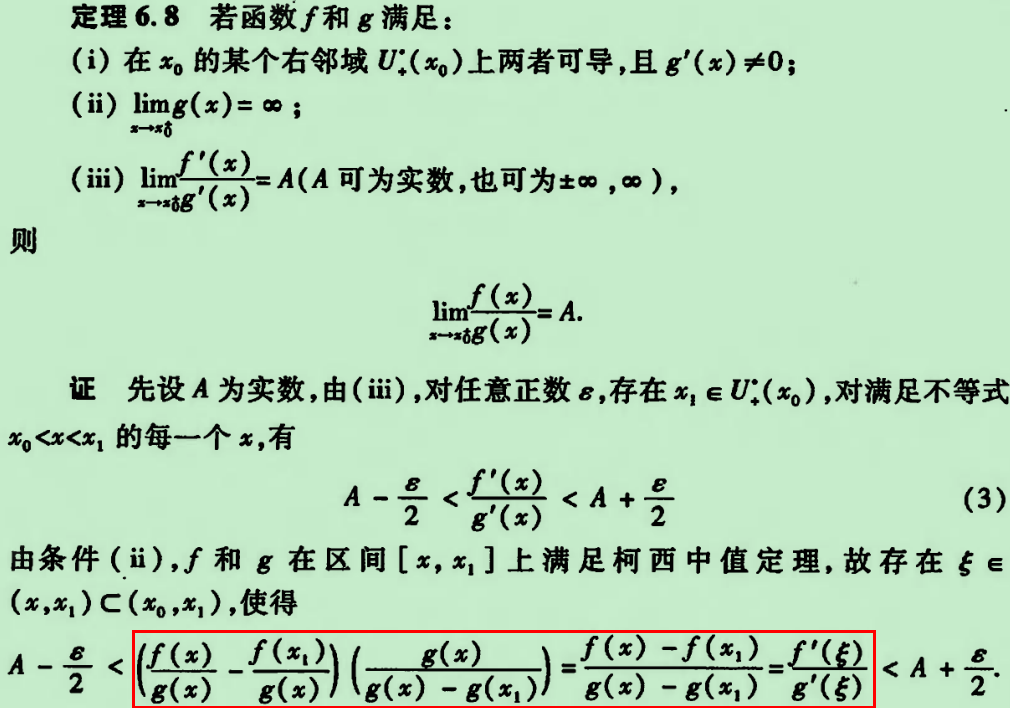# 洛必达法则的证明探讨

0/0型洛必达法则 1 （L’Hospital’s Rule: 0/0 case）：在区间(a, b)上，f(x)和g(x)都可导、 $None$ $None$ ，如果 $$\lim_{x \rightarrow a^{+}}\frac{f^{'}(x)}{g^{'}\left( x \right)} = L$$ ，那么

\lim_{x \rightarrow a^{+}}\frac{f\left( x \right)}{g\left( x \right)} = \lim_{x \rightarrow a^{+}}\frac{f^{'}\left( x \right)}{g^{'}\left( x \right)} = L.

\frac{f(x) - f(a)}{g\left( x \right) - g\left( a \right)} = \frac{f'(c)}{g^{'}\left( c \right)}

\frac{f(x)}{g\left( x \right)} = \frac{f'(c)}{g^{'}\left( c \right)}

$None$ 时，因为c在(a, x)上，所以 $None$ ，又因为 $$\lim_{x \rightarrow a}\frac{f^{'}(x)}{g^{'}\left( x \right)} = L$$ ，所以

\lim_{x \rightarrow a^{+}}\frac{f\left( x \right)}{g\left( x \right)} = \lim_{x \rightarrow a^{+}}\frac{f^{'}\left( c \right)}{g^{'}\left( c \right)} = \lim_{c \rightarrow a^{+}}\frac{f^{'}\left( c \right)}{g^{'}\left( c \right)} = \lim_{x \rightarrow a^{+}}\frac{f^{'}\left( x \right)}{g^{'}\left( x \right)} = L.

1. 证明开头处补充定义f(a)=g(a)=0不会影响 $$\lim_{x \rightarrow a^{+}}\frac{f\left( x \right)}{g\left( x \right)}$$ 吗？不会 2 ！函数在 $None$ 时的极限和x=a处的值无关（与函数在x=a处近旁的值有关），改变函数在x=a处的值并不会影响函数在 $None$ 时的极限。为了更形象地理解这个特性，各位请随便定义下图中 $None$ $None$ 于x=0处的值，然后再观察一下这种改变是否会影响到 $$\lim_{x \rightarrow 0^{+}}\frac{\text{sinx}}{- 0.5x}$$ ？（ $$\lim_{x \rightarrow 0^{+}}\frac{\text{sinx}}{- 0.5x} = - 2$$ ）。总之，f(x)和g(x)在a处是否有定义或取什么值并不影响 $$\lim_{x \rightarrow a^{+}}\frac{f\left( x \right)}{g\left( x \right)}$$ ，因此0/0型洛必达法则的成立条件也就不要求f(x)和g(x)在a处连续了，上面的证明过程中也才可以补充定义f(a)=g(a)=0来进行证明。补充这个定义仅仅只是让我们可以在证明过程中使用柯西中值定理，它不会对 $$\lim_{x \rightarrow a^{+}}\frac{f\left( x \right)}{g\left( x \right)}$$ 是否存在或是值是多少有影响，所以它并未构成0/0型洛必达法则的成立条件之一。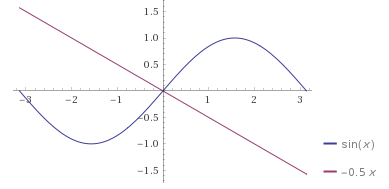2. 用和上面类似的方法不难证明0/0型洛必达法则在 $None$ 时也成立，进而可得到在 $None$ 时也成立。

3. 如果 $$\lim_{x \rightarrow a^{+}}\frac{f^{'}\left( x \right)}{g^{'}\left( x \right)}$$ 仍然是0/0型，那么在确认 $None$ $None$ 满足0/0型洛必达法则成立条件的情况下仍可得出 $$\lim_{x \rightarrow a^{+}}\frac{f^{'}\left( x \right)}{g^{'}\left( x \right)} = \lim_{x \rightarrow a^{+}}\frac{f^{''}\left( x \right)}{g^{''}\left( x \right)}$$ ，在需要的时候，这一过程可以再继续下去 3

∞/∞型洛必达法则 4 （L’Hospital’s Rule: ∞/∞ case）：在区间(a, b)上，f(x)和g(x)都可导、 $None$ $None$ ，如果 $$\lim_{x \rightarrow a^{+}}\frac{f^{'}(x)}{g^{'}\left( x \right)} = L$$ ，那么

\lim_{x \rightarrow a^{+}}\frac{f\left( x \right)}{g\left( x \right)} = \lim_{x \rightarrow a^{+}}\frac{f^{'}\left( x \right)}{g^{'}\left( x \right)} = L.

• 常庆哲、史济怀，《数学分析教程》上册（2003），p179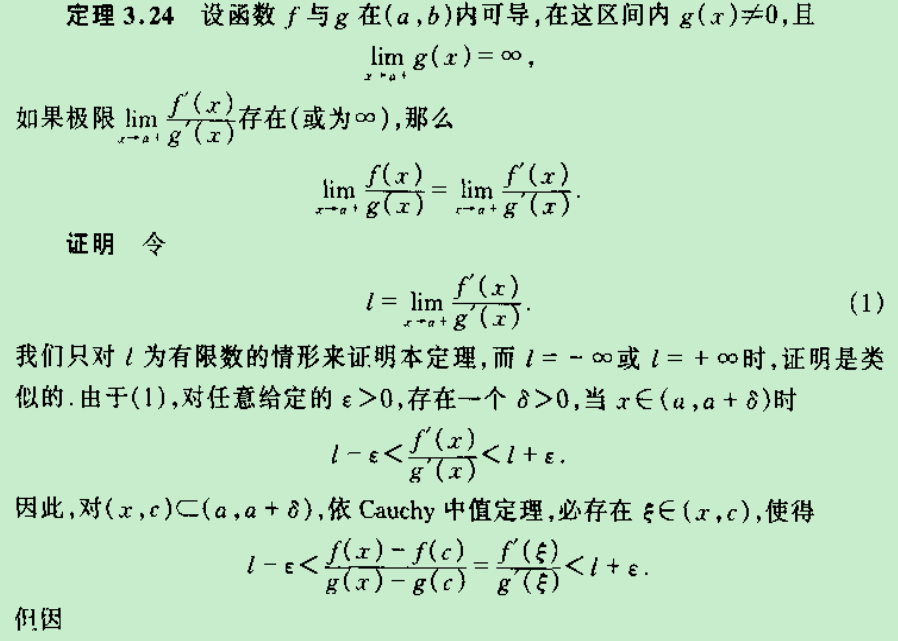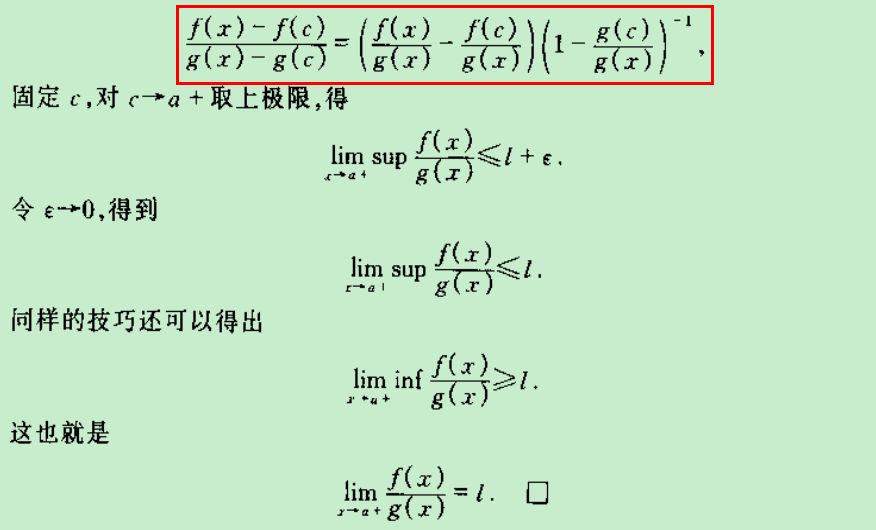• 陈纪修、於崇华、金路《数学分析》上册，第二版，P125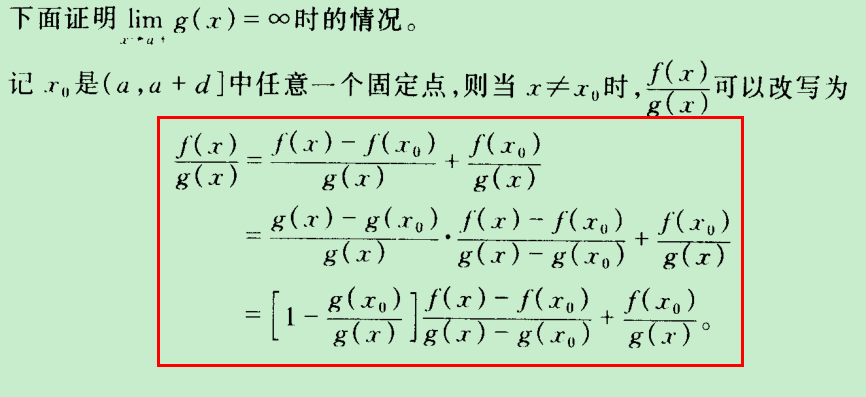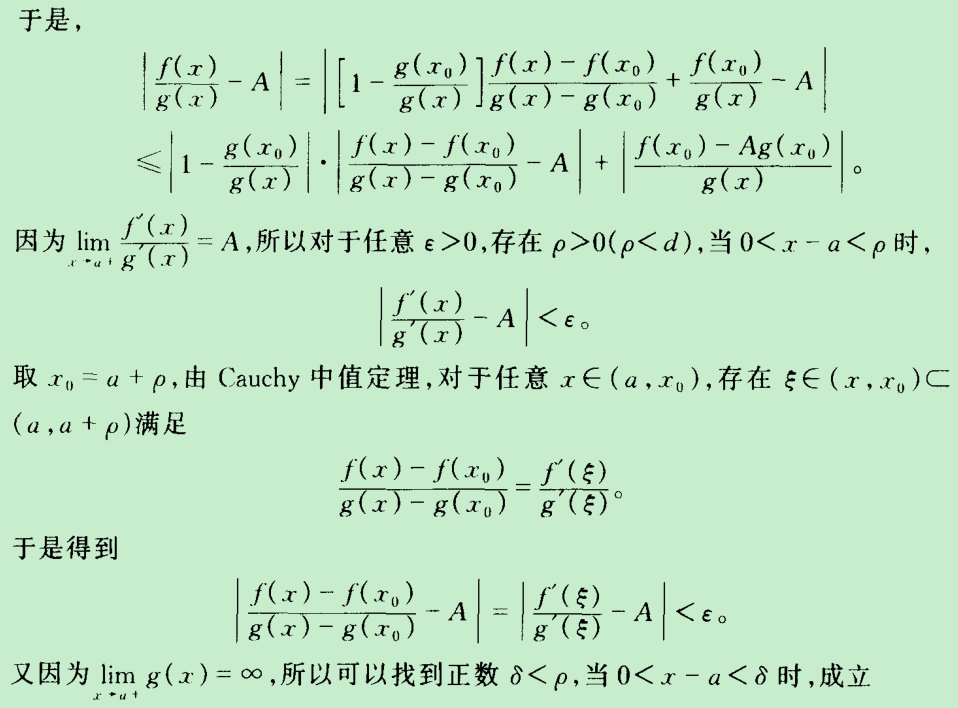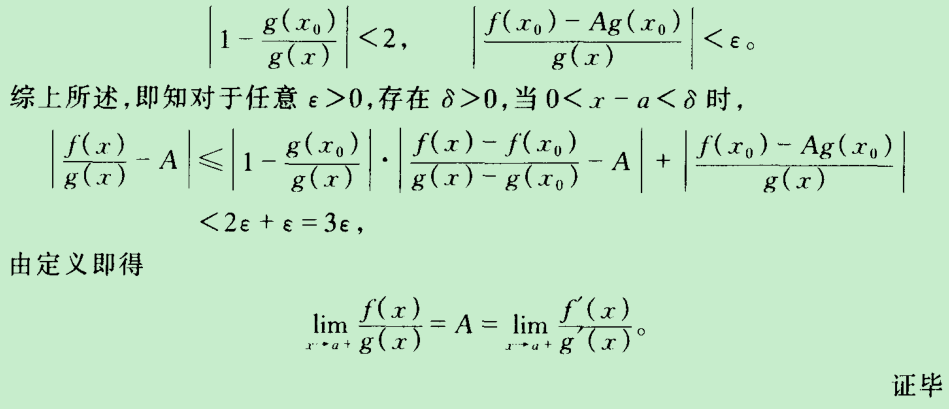• 华东师范大学数学系，《数学分析》上册，第四版，P132